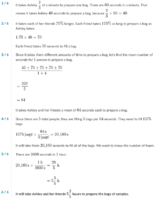# Preparing Bags Problem

#### Riddle Crush

##### New member
I came across a question on Khan Academy that confuses me. The question was something like this:

Ashley takes 40 seconds to prepare one bag. She receives help from 4 friends in preparing bags. Each of her friends take 70 seconds to prepare one bag. How many hours will it take them all to prepare 1575 bags?

To answer this question, I used this method:
Step 1: Find the lowest common multiple of 40 and 70, which is 280.
Step 2: In 280 seconds, Ashley can prepare 7 bags, and each of her 4 friends can prepare 4 bags. That's 23 bags in total.
Step 3: The average time taken between all 5 of them in preparing one bag is 280 ÷ 23 = 12.17391304 seconds
Step 4: The total time taken in hours in preparing 1575 bags is 1575 x 12.17391304 ÷ 3600 = 5.326086957 hours

Khan Academy told me this was the wrong answer, and showed the apparently correct method of working out the answer:
Step 1: Find the average time it takes one of them to prepare one bag: (40 + (4 x 70)) ÷ 5 = 64 seconds
Step 2: Since there are 5 workers, it's 5 times faster, so 64 ÷ 5 = 12.8 seconds
Step 3: The total time taken in hours in preparing 1575 bags is 1575 x 12.8 ÷ 3600 = 5.6 hours.

I later realised an alternative to my method:
Follow steps 1 and 2 of my method.
Step 3: 1575 ÷ 23 = 68 with a remainder of 11, so 68 bouts, each lasting 280 seconds, produces 1564 bags.
Step 4: The remaining 11 bags can be produced in 140 seconds. In this time, each of Ashley's 4 friends can prepare 2 bags, and Ashley can prepare 3 bags and finish 20 seconds before the others.
Step 5: 68 bouts of 280 seconds is 19,040 seconds
Step 6: 19,040 seconds plus the remaining 140 seconds is 19,180 seconds
Step 7: 19,180 seconds ÷ 3600 = 5.327777778 hours

To me, the third method seems like it should produce the correct result, but Khan Academy had different ideas. And the first two methods seem to me like they should both produce the same result, but they don't. Can anyone explain this please?

#### lev888

##### Full Member
Ashley's rate: 1/40 bag/sec
Friend's rate: 1/70 bag/sec
Combined rate: 1/40 + 4/70 = 23/280 bag/sec
1575 bags will take 1575/(23/280) sec = (1575*280)/23 sec = 19,173.913 sec = 5.326 hours

#### Jomo

##### Elite Member
In 280 seconds, Ashley can prepare 7 bags, and each of her 4 friends can prepare 4 bags. That's 23 bags in 280 seconds.
So the question is how many 23 bags do we need to make 1575 bags. That will be 1575/23 periods which each take 280 seconds. Now (1575/23)*280 =441000/23 seconds. Divide by 3600 yields ~5.33 hrs

#### Dr.Peterson

##### Elite Member
Simple explanation: Khan is wrong!

Averaging times doesn't work; you have to average rates instead. For example, if I take 1 hour to do a job, and you take 9 hours, our average time is 5 hours, so Khan would apparently say we take 2.5 hours per job together. But in 9 hours we will do 10 jobs together, so our combined rate is 10/9 jobs per hour, which is 9/10 hour per job.

Can you show us where they say this?

#### Riddle Crush

##### New member
Thanks for the response guys. It's encouraging to know that you all seem to be thinking along the same lines as me and that I may have the correct maths.

Dr.Peterson, yes it seems as though averaging rates is better than averaging times. I think that the question was the last question in the set of 4 questions shown here:

I assume that you will see the right question at question 4. If you happen to have completed this set of questions before, then you may not see the same question that I am asking about.

#### Dr.Peterson

##### Elite Member
Here is an image of what they say:That, of course, is wrong. We can check it, by finding how many bags they can prepare in that time:

Ashley does one bag per 40 seconds, so in 20,160 seconds she does 20,160/40 = 504 bags.
Each friend does one bag per 70 seconds, so in 20,160 seconds she does 20,160/70 = 288 bags.
Altogether, they do 504 + 4(288) = 1656 bags. That is not 1575; it takes less time than that.

How about checking our answer, which was (to be exact) (1575*280)/23 seconds:

Ashley does one bag per 40 seconds, so in (1575*280)/23 seconds she does (1575*280)/(23*40) = 441000/920 = 479.35 bags.
Each friend does one bag per 70 seconds, so in (1575*280)/23 seconds she does (1575*280)/(23*70)= 441000/1610 = 273.91 bags.
Altogether, they do 479.35 + 4(273.91) = 1574.99 bags. That is (apart from rounding) 1575.

So, yes -- you are right (and your final method just makes it more precise), and they are wrong.

•Subhotosh Khan

#### Riddle Crush

##### New member
Thanks for posting that Dr.Peterson. It's nice to know I was right.